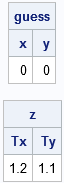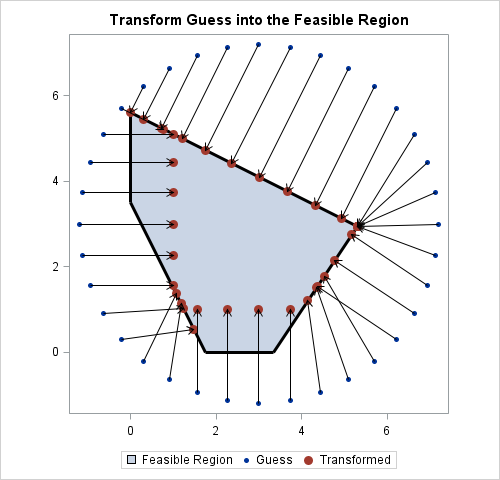Most numerical optimization routines require that the user provides an initial guess for the solution. I have previously described a method for choosing an initial guess for an optimization, which works well for low-dimensional optimization problems. Recently a SAS programmer asked how to find an initial guess when there are linear constraints and bounds on the parameters. There are two simple approaches for finding an initial guess that is in the feasible region. One is the "shotgun" approach in which you generate many initial guesses and evaluate each one until you find one that is feasible. The other is to use the NLPFEA subroutine in SAS/IML, which takes any guess and transforms it into a feasible point in the linearly constrained region.

### The NLPFEA subroutine in SAS/IML software

The NLPFEA routine returns a point in the feasible region from an arbitrary starting guess. Suppose the problem has p (possibly bounded) parameters, and the feasible region is formed by k > 0 additional linear constraints. Then you can represent the feasible regions by a (k+2) x (p+2) matrix, which is the representation that is used for linear programming and constrained nonlinear optimizations. The first row specifies the lower bounds for the parameters and the second row specifies the upper bounds. (Missing values in the first p columns indicate that a parameter is not bounded.) The remaining k rows indicate linear constraints. For example, the following matrix defines a pentagonal region for two parameters. You can call the NLPFEA subroutine to generate a point that satisfies all constraints:

```proc iml; con = { 0 0 . ., /* param min */ 10 10 . ., /* param max */ 3 -2 -1 10, /* 3*x1 + -2*x2 LE 10 */ 5 10 -1 56, /* 5*x1 + 10*x2 LE 56 */ 4 2 1 7 }; /* 4*x1 + 2*x2 GE 7 */   guess = {0 0}; /* arbitrary p-dimensional point */ call nlpfea(z, guess, con); /* x0 is feasible point */ print guess[c={"x" "y"}], z[c={"Tx" "Ty"}];```The output shows that the guess (x,y) = (0,0) was not feasible, but the NLPFEA routine generated the transformed point T(x,y) = (1.2, 1.1), which is feasible.

It is interesting to visualize the NLPFEA subroutine. The following SAS/IML statements create 36 initial guesses that are distributed uniformly on a circle around the feasible region. For each guess, the program transforms the guess into the feasible region by calling the NLPFEA subroutine. The initial and transformed points are saved to a SAS data set and visualized by using PROC SGPLOT:

```NumPts = 36; twopi = 2*constant('pi'); x=.; y=.; Tx=.; Ty=.; create feasible var {"x" "y" "Tx" "Ty"}; do i = 1 to NumPts; x = 2.5 + 5*cos((i-1)/NumPts * twopi); /* guess on circle */ y = 2.5 + 5*sin((i-1)/NumPts * twopi); call nlpfea(feasPt, x||y, con); /* transform into feasible */ Tx = feasPt; Ty = feasPt; append; end; close;```The graph visualizes the result. The graph shows how each point on the circle is transformed into the feasible region. Some points are transformed into the interior, but many are transformed onto the boundary. You can see that the transformed point always satisfies the linear constraints.

### SAS/IML automatically finds feasible points

Before I finish, I want to point out that the nonlinear programming (NLP) subroutines in SAS/IML software rarely require you to call the NLPFEA subroutine explicitly. When you call an NLP routine for a linearly constrained optimization and provide a nonfeasible initial guess, the NLP routine internally calls the NLPFEA routine. Consequently, you might see the following NOTE displayed in the SAS log: NOTE: Initial point was changed to be feasible for boundary and linear constraints. For example, run the following program, which provides a nonfeasible initial guess to the NLPNRA (Newton-Raphson) subroutine.

```start func(x); x1 = x[,1]; x2 = x[,2]; return ( -(x1-3)##4 + -(x2-2)##2 + 0.1*sin(x1#x2)); finish;   opt = {1, /* find maximum of function */ 3}; /* print a little bit of output */ x0 = {0 0}; call nlpnra(rc, x_Opt, "func", x0, opt) blc=con;```

In this program, the NLPNRA subroutine detects that the guess not feasible. It internally calls NLPFEA to obtain a feasible guess and then computes an optimal solution. This is very convenient for programmers. The only drawback is that you don't know the initial guess that produced the optimal solution. However, you can call the NLPFEA subroutine directly if you want to obtain that information.

### Summary

In optimization problems that have linear and boundary constraints, most optimization routines require an initial guess that is in the feasible region. The NLPFEA subroutine enables you to obtain a feasible point from an arbitrary initial guess. You can then use that feasible point as an initial guess in a built-in or user-defined optimization routine. However, for the built-in NLP subroutines, you can actually skip the NLPFEA call because the NLP subroutines internally call NLPFEA when you supply a nonfeasible initial guess.

ShareDistinguished Researcher in Computational Statistics

Rick Wicklin, PhD, is a distinguished researcher in computational statistics at SAS and is a principal developer of SAS/IML software. His areas of expertise include computational statistics, simulation, statistical graphics, and modern methods in statistical data analysis. Rick is author of the books Statistical Programming with SAS/IML Software and Simulating Data with SAS.

1.anand seeram on

Hi Rick,
Can you share the code for the graph of feasible region?

Thanks.

•2.•